# Lesson 8 homework assignments

(Karthik Kannan) #22

Hey @mattobrien415,

you need to pass the conv block a shape of (?,72,72,3). The way to do it is
inp_shape = arr_lr.shape[1:]
In that way you’ll pass a Tensor with shape(?,72,72,3) instead of a Tensor with shape (?,19340,72,72,3).

Hope that helps.EDIT: GRAMMAR!

(Constantin) #23

I saw this blog post on Hackernoon which explains GANs “in plain English” as they put it. There is actually a sort of conversation between the generator and the discriminator as in between student and teacher. At the bottom it has a link to a presentation by Ian Goodfellow on GANs where he reviews current applications of GANs in the scientific literature. He makes references to some of the publications we got as reading assignments for lesson 8 (e.g. Ledig et al).

(Jeremy Howard (Admin)) #24

Just in case anyone is still stuck with creating the filename list, here’s how I did it:

``````fnames = list(glob.iglob(path+'train/*/*.JPEG'))
pickle.dump(fnames, open(dpath+'fnames.pkl', 'wb'))``````

(mattobrien415) #25

In our implementation, we need to define an object that will allow us to separately access the loss function and gradients of a function, since that is what scikit-learn’s optimizers require.

Should this say scipy’s optimizers? We are using fmin_l_bfgs_b, which appears to be from scipy not sklearn.

(Even Oldridge) #26

I’m just going to throw this out here because it’s bugging me mentally and preventing me from making the type of style layers that I want: something about the training process to recreate the input bothers me…

If convolutions are shift invariant then why does the training of a system to reproduce those layers result in the same (or almost same) image? Is it the chaining of complex convolutions one after the other? Has the net really learned the relationship between a given point in the image and all other points within that single layer?

If that’s the case and I’m looking to create style layers with some structure but not the entire structure of the image does that mean I want to do so on earlier layers?

(Matthew Kleinsmith) #27

While trying to answer Even’s question I realized I lacked understanding.

I don’t know how to produce the first style reconstruction of this figure:

## The first style reconstruction of the figure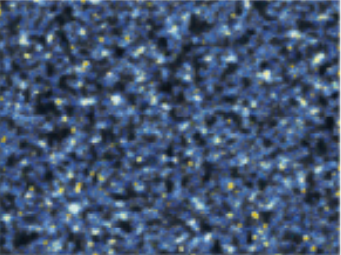## Some code

``````# preprocess the style image
style_image = Image.open('data/starry_night.jpg')
style_image = style_image.resize(np.divide(style_image.size, 3.5).astype('int32'))
style_arr = preproc(np.expand_dims(style_image, 0)[:,:,:,:3])
shp = style_arr.shape

# create the style model
model = VGG16_Avg(include_top=False, input_shape=shp[1:])
outputs = {l.name: l.output for l in model.layers}
layers = [outputs['block{}_conv1'.format(o)] for o in [1, 2]]
layers_model = Model(model.input, layers)
targs = [K.variable(o) for o in layers_model.predict(style_arr)]

# define the style loss
loss = sum(style_loss(l1, l2) for l1,l2 in zip(layers, targs))
style_fn = K.function([model.input], [loss]+grads)
evaluator = Evaluator(style_fn, shp)

# extract the style
x = rand_img(shp)
iterations = 10
x = solve_image(evaluator, iterations, x)
``````

## The code produces this style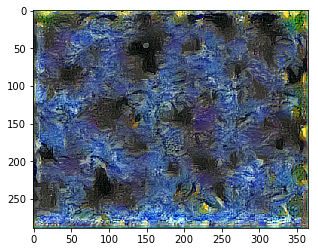## How can we change the code to produce the following style?## Attempt 1

Change this line:

``````layers = [outputs['block{}_conv1'.format(o)] for o in [1, 2]]
``````

to

``````layers = [outputs['block{}_conv1'.format(o)] for o in [1, 1]]
``````

This should look at block1_conv1 twice, which should be equivalent to looking at it once in terms of optimization.

However, this is the result: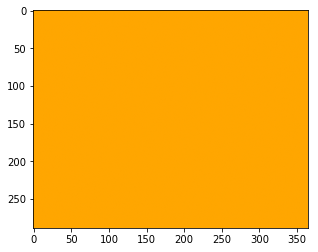And here are the losses:

``````Current loss value:  1350.84924316
Current loss value:  1350.84936523
[...]
Current loss value:  1350.84936523
Current loss value:  1350.84936523
``````

## Attempt 2

Change these lines:

``````layers = [outputs['block{}_conv1'.format(o)] for o in [1, 1]]
layers_model = Model(model.input, layers)
targs = [K.variable(o) for o in layers_model.predict(style_arr)]

# define the style loss
loss = sum(style_loss(l1, l2) for l1,l2 in zip(layers, targs))
``````

to

``````layer = model.get_layer('block1_conv1').output
layer_model = Model(model.input, layer)
targ = K.variable(layer_model.predict(style_arr))

# define the style loss
loss = style_loss(layer, targ)
``````

Results: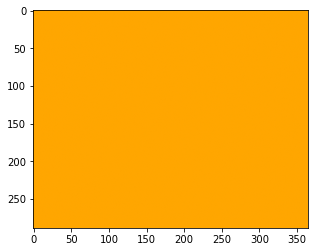``````Current loss value:  675.424560547
Current loss value:  675.424682617
[...]
Current loss value:  675.424682617
Current loss value:  675.424682617
``````

## Starry night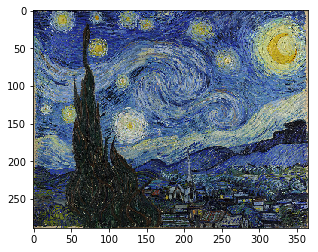## Starry night preprocessed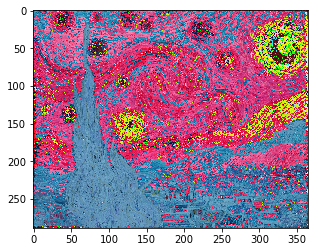## block1_conv1 activations of Starry night

You can open the image in a new tab to see a bigger version of it.

## Random image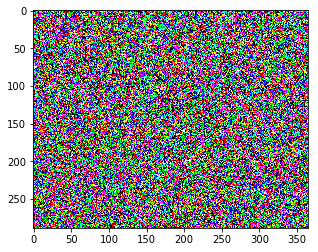## Gram matrices of block1_conv1 activations of random image

(Jeremy Howard (Admin)) #28

Good point - oops!

(Jeremy Howard (Admin)) #29

The problem is that your random image doesn’t have enough variance, so the gradient points towards a constant. If you remove the ‘/100’ from the random generator, you’ll get this (at least, I did):

(Matthew Kleinsmith) #30

Thank you. That was it.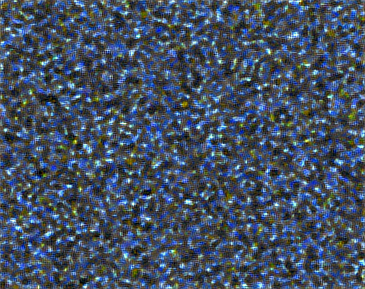Replaced:

``````rand_img = lambda shape: np.random.uniform(-2.5, 2.5, shape) / 100
``````

With:

``````rand_img = lambda shape: np.random.uniform(-2.5, 2.5, shape)
``````

(Jeremy Howard (Admin)) #31

One idea which I think can help all image generation is to blur the random noise. Here’s what this style image looks like with blurred noise as the starting point:

It’s a little less pixelated, I think.

(Sourav Dey) #32

Random aside, I made these block diagrams to help me understand what is going on in the image transfer. Maybe they’re helpful to someone in understanding what is happening better. I also boxed up some of the key questions I have that i’ll be exploring later.

## Gradient Descent to do Image Style Transfer

(Sourav Dey) #33

Great post! I’m gonna totally follow this advice(David Gutman) #34

Just a few images from Brooklyn…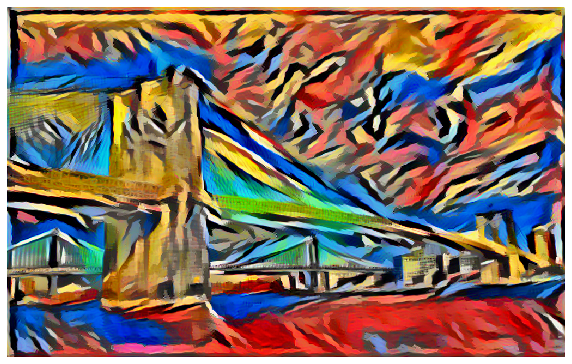(David Gutman) #35

(Jeremy Howard (Admin)) #36

@davecg you’re really good at selecting nicely matching images!(David Gutman) #37

Saw this paper and wanted to try it out:

The histogram matching thing didn’t work that well for me, but luminance only style transfer worked pretty well.

``````import os
from scipy.misc import imread, imsave, imresize
from skimage.color import luv2rgb, rgb2luv

def save_luminance(fp, suffix='lum', img_format='png'):
# save luminance from image, need to do this for style and content
# then use style transfer on luminance images
op = '{}_{}.{}'.format(os.path.splitext(fp), suffix, img_format)
lum = rgb2luv(img)[...,0]
imsave(op, lum, format=img_format)

def combine_luminance(a, fp):
# add uv from original file to output of luminance style transfer
if a.ndim > 2:
# grayscale
a = a.mean(axis=-1)
assert a.ndim == 2, 'Can only accept 2D or 3D data.'
img = imresize(imread(fp), (a.shape, a.shape, 3))
luv = rgb2luv(img)

# need to rescale

mean_a = a.mean()
std_a = a.std()
mean_lum = luv[...,0].mean()
std_lum = luv[...,0].std()
adjusted_a = (a - mean_a)*(std_lum/std_a) + mean_lum

return luv2rgb(luv)
``````

starry night style with luminance only style transfer:

Curating Lesson 8 experiments
(Mariya) #38

Your theory seems to be right. I tried to turn a fish into an anime fish with Sailor Moon style, but ran into the same poor application of style issue. When you say “start with the original image as the initial condition” to get the better application of Dr. Seuss, what does this mean in implementation?

(nima) #39

Is it just me or is this supposed to run on the CPU?

It’s taking a long time for solve_image to run for me. Am I doing something wrong in terms of my setup that doesn’t enable GPU?

(Kishore P. V.) #40

Here is my results: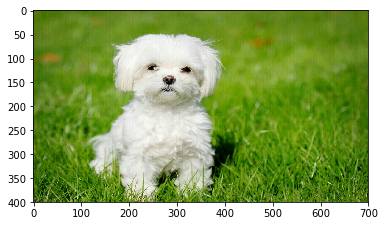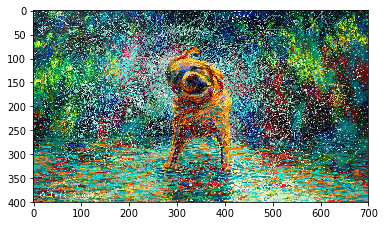(Kishore P. V.) #41